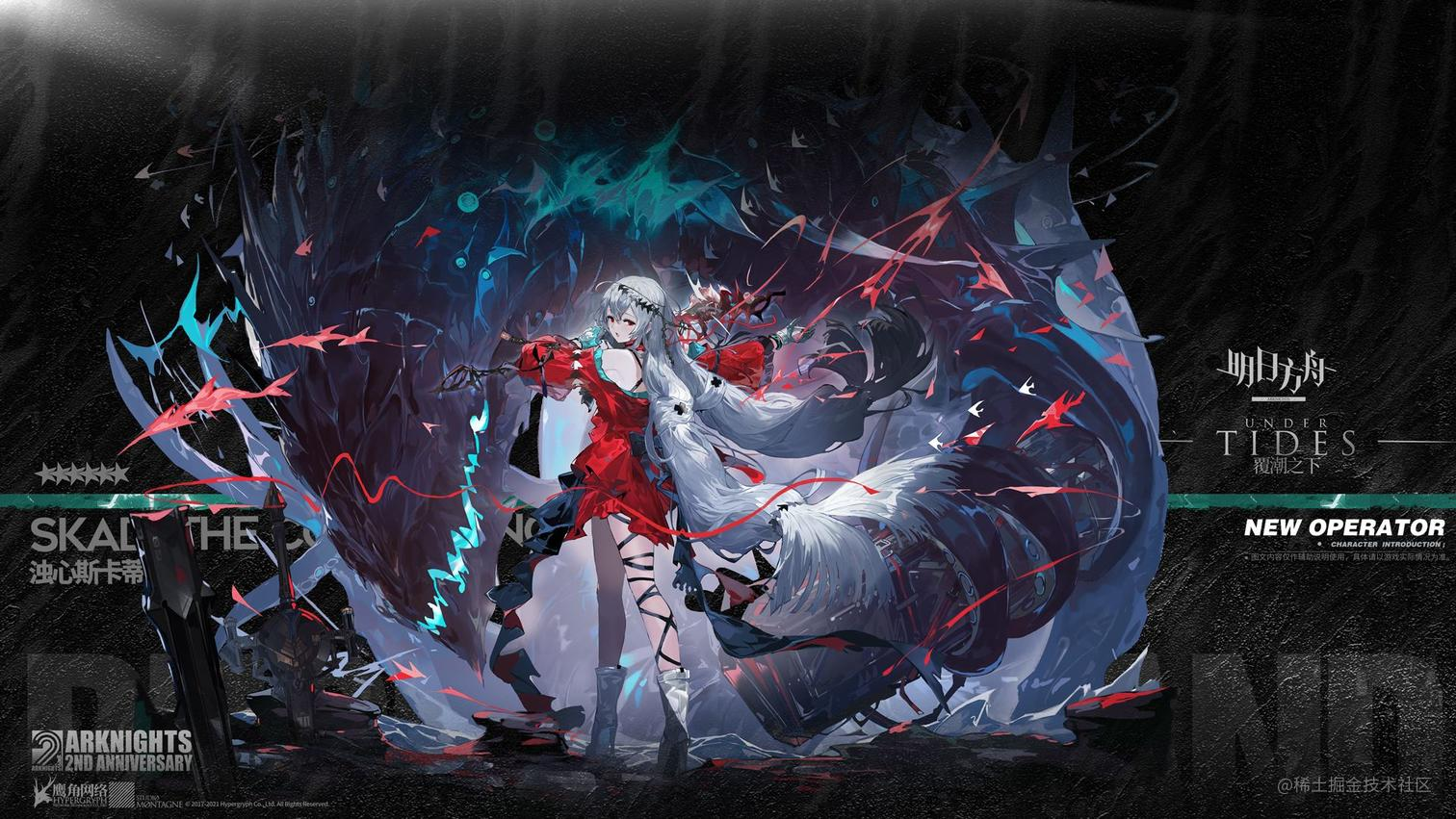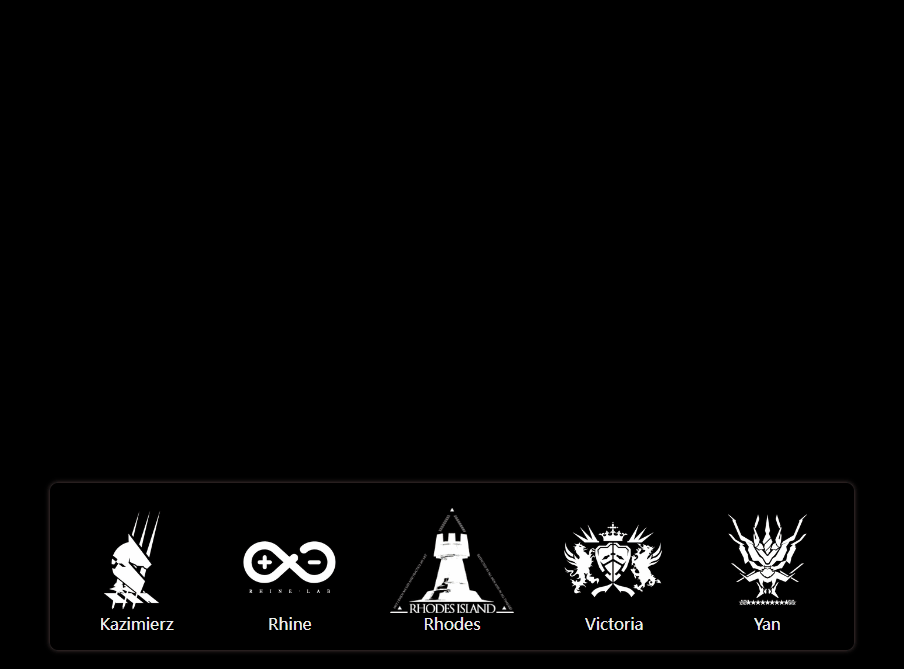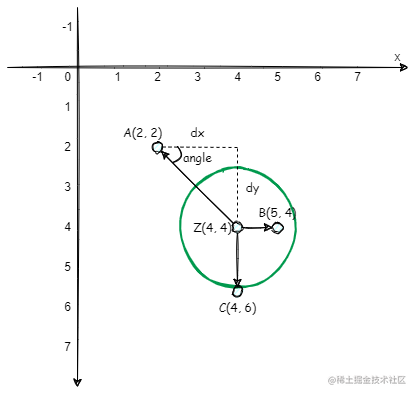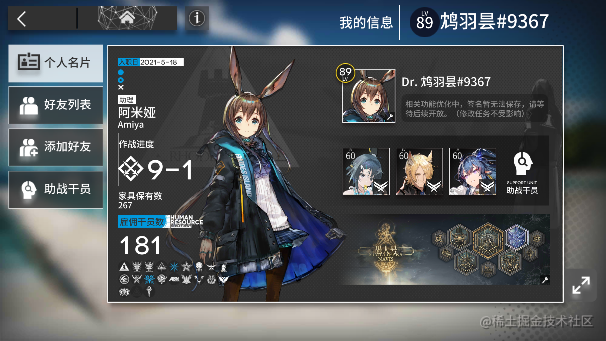# 【Canvas实战】仿明日方舟Logo粒子动画 vue3+ts## 前言🎀## 最终效果

### 动画### 源码

github.com/XIwE1/ark-p…

1. Particle： 记录粒子位置、颜色、大小、动画耗时 和 x/y 方向上的移动速度，提供绘制粒子方法`draw`、更新方法`update`、替换方法`change`
2. LogoImg： 记录图片解析后的粒子数组信息`particleData`
3. ParticleCanvas： 记录目标画布、画布中的粒子数组和鼠标在画布中的位置，提供绘制画布方法`drawCanvas`、改变粒子数组方法`changeImg`

• 实例化一个`ParticleCanvas`对象`prtCanvas`
• 点击某个图片`clickLogo`时调用`prtCanvas.changeImg(particleData)`方法传入其粒子数组信息。
• 首次 changeImg，直接赋值
• 非首次，对比粒子数组 移除/生成粒子，并随机映射

• 鼠标在实例对象`prtCanvas`对应的画布移动时触发`mousemove`回调，根据回调参数重新计算鼠标位置`mouseX/mouseY`
• `prtCanvas`的绘制画布方法`drawCanvas`一直随着事件循环在执行，`drawCanvas`中遍历画布粒子数组并调用每一项的`update`方法并传入重新计算后的`mouseX/mouseY`
• `particle.update`中又根据距离和设置好的引力/斥力重新计算`vx/vy`...
``````this.ParticleArr.forEach((particle) => {
particle.update(this.mouseX, this.mouseY);
particle.draw();
});

Particle 的 draw 方法符合面向对象的写法是接收一个 content 上下文参数，图方便就直接读取了 😁

## 分析

1. 解析图片转换为粒子
2. 绘制时添加动画
3. 根据鼠标位置对粒子进行排斥

## 像素操作

canvas提供了 绘制图片 和 获取图片像素 的方法，但在绘制图片或者获取图片信息用于操作之前，首先要获取目标图片源

``````    ......
let img = new Image();
img.src = src;
// canvas 获取粒子位置数据
// 获取图片像素数据
const tmp_canvas = document.createElement("canvas"); // 创建一个空的canvas
const tmp_ctx = tmp_canvas.getContext("2d");

tmp_ctx?.drawImage(img, 0, 0, imgW, imgH); // 将图片绘制到canvas中
const imgData = tmp_ctx?.getImageData(0, 0, imgW, imgH).data; // 获取像素点数据
tmp_ctx?.clearRect(0, 0, width, height);
};
......

`ImageData``data`属性为 Uint8ClampedArray 类型的一维数组，包含了指定区域里每个像素点的RGBA格式的整型数据，范围在0至255之间（包括255）。

## canvas动画

canvas的动画主要是通过 在一些定时方法中去执行重绘操作实现的。

canvas实现动画的过程通常是 清理->绘制->清理->绘制...  不断重复的过程。

## 实现

### 生成粒子/绘制画布

#### 粒子类

``````class Particle {
totalX: number; // 粒子x轴的目标位置
totalY: number; // 粒子y轴的目标位置
r: number; // 粒子的半径
color: number[]; // 粒子的颜色
opacity: number; // 粒子的透明度
constructor(totalX: number, totalY: number, time: number, color: number[]) {
// 目标位置dx、dy，总耗时time
this.totalX = totalX;
this.totalY = totalY;
// 设置粒子的颜色和半径
this.r = 1.2;
this.color = [...color];
this.opacity = 0;
}
// 在画布中绘制粒子
draw() {}
// 更新粒子
update() {}
// 切换粒子
change() {}
}

#### 筛选像素

``````......
// 获取图片像素数据
......
const imgData = tmp_ctx?.getImageData(0, 0, imgW, imgH).data; // 获取像素点数据
tmp_ctx?.clearRect(0, 0, width, height);

// 筛选像素点
for (let y = 0; y < imgH; y += 5) {
for (let x = 0; x < imgW; x += 5) {
// 像素点的索引
const index = (x + y * imgW) * 4;
// 在数组中对应的值
const r = imgData![index];
const g = imgData![index + 1];
const b = imgData![index + 2];
const a = imgData![index + 3];
const sum = r + g + b + a;
// 筛选条件
if (sum >= 100) {
const particle = new Particle(x, y, animateTime, [r, g, b, a]);
this.particleData.push(particle);
}
}
}
};
......

#### 创建粒子

1. `x、y`属性表示粒子当前位置
2. `mx、my`属性表示粒子需要移动的距离
3. `vx、vy`属性表示粒子在方向上的移动速度
4. `time`属性表示粒子过渡动画所耗时间
5. `update`方法在粒子更新时调用，在其中动态计算`mx、my、vx、vy`
6. `draw`方法在画布中绘制粒子
``````class Particle {
x: number; // 粒子x轴的初始位置
y: number; // 粒子y轴的初始位置
totalX: number; // 粒子x轴的目标位置
totalY: number; // 粒子y轴的目标位置
mx?: number; // 粒子x轴需要移动的距离
my?: number; // 粒子y轴需要移动的距离
vx?: number; // 粒子x轴移动速度
vy?: number; // 粒子y轴移动速度
time: number; // 粒子移动耗时
r: number; // 粒子的半径
color: number[]; // 粒子的颜色
opacity: number; // 粒子的透明度
constructor(totalX: number, totalY: number, time: number, color: number[]) {
// 设置粒子的初始位置x、y，目标位置dx、dy，总耗时time
this.x = (Math.random() * width) >> 0;
this.y = (Math.random() * height) >> 0;
this.totalX = totalX;
this.totalY = totalY;
this.time = time;
// 设置粒子的颜色和半径
this.r = 1.2;
this.color = [...color];
this.opacity = 0;
}
/** 更新粒子
* @param {number} mouseX 鼠标X位置
* @param {number} mouseY 鼠标Y位置
*/
update(mouseX?: number, mouseY?: number) {
// 设置粒子需要移动的距离
this.mx = this.totalX - this.x;
this.my = this.totalY - this.y;
// 设置粒子移动速度
this.vx = this.mx / this.time;
this.vy = this.my / this.time;
this.x += this.vx;
this.y += this.vy;
// 随着移动不断增加透明度
if (this.opacity < 1) this.opacity += opacityStep;
}
// 在画布中绘制粒子
draw() {
context.beginPath()
context.value!.fillStyle = `rgba(\${this.color.toString()})`;
context.value!.arc(this.x, this.y, this.r * 2, 0, 2 * Math.PI);
context.value!.fill();
context.closePath()
}
}

#### 绘制画布

``````/** Logo图片类 */
class LogoImg {
src: string;
name: string;
particleData: Particle[]; // 用于保存筛选后的粒子
constructor(src: string, name: string) {
this.src = src;
this.name = name;
this.particleData = [];
let img = new Image();
img.crossOrigin = '';
img.src = src;
// canvas 获取粒子位置数据
// 获取图片像素数据
const tmp_canvas = document.createElement("canvas"); // 创建一个空的canvas
const tmp_ctx = tmp_canvas.getContext("2d");
const imgW = width;
const imgH = ~~(width * (img.height / img.width));
tmp_canvas.width = imgW;
tmp_canvas.height = imgH;
tmp_ctx?.drawImage(img, 0, 0, imgW, imgH); // 将图片绘制到canvas中
const imgData = tmp_ctx?.getImageData(0, 0, imgW, imgH).data; // 获取像素点数据
tmp_ctx?.clearRect(0, 0, width, height);

// 同上筛选像素点
};
}
}

// 画布类
class ParticleCanvas {
canvasEle: HTMLCanvasElement;
ctx: CanvasRenderingContext2D;
width: number;
height: number;
ParticleArr: Particle[];
constructor(target: HTMLCanvasElement) {
this.canvasEle = target;
this.ctx = target.getContext("2d") as CanvasRenderingContext2D;
this.width = target.width;
this.height = target.height;
this.ParticleArr = [];
}
// 改变画布数据源
changeImg(img: LogoImg) {
this.ParticleArr = img.particleData.map(
(item) =>
new Particle(item.totalX, item.totalY, animateTime, item.color)
);
}
// 画布绘制方法
drawCanvas() {
this.ctx.clearRect(0, 0, this.width, this.height);
this.ParticleArr.forEach((particle) => {
particle.update();
particle.draw();
});
window.requestAnimationFrame(() => this.drawCanvas());
}
}

### 切换动画

``````  // 改变图片 如果已存在图片则进行额外切换操作
changeImg(img: LogoImg) {
if (this.ParticleArr.length) {
// 如果当前粒子数组大于新的粒子数组 删除多余的粒子
let newPrtArr = img.particleData;
let newLen = newPrtArr.length;
let arr = this.ParticleArr;
let oldLen = arr.length;

// 调用change修改已存在粒子
for (let idx = 0; idx < newLen; idx++) {
const { totalX, totalY, color } = newPrtArr[idx];
if (arr[idx]) {
// 找到已存在的粒子 调用change 接收新粒子的属性
arr[idx].change(totalX, totalY, color);
} else {
arr[idx] = new Particle(totalX, totalY, animateTime, color);
}
}

if (newLen < oldLen) this.ParticleArr = arr.splice(0, newLen);
let tmp_len = arr.length;
// 随机打乱粒子最终对应的位置 使切换效果更自然
while (tmp_len) {
// 随机的一个粒子 与 倒序的一个粒子
let randomIdx = ~~(Math.random() * tmp_len--);
let randomPrt = arr[randomIdx];
let { totalX: tx, totalY: ty, color } = randomPrt;

// 交换位置
randomPrt.totalX = arr[tmp_len].totalX;
randomPrt.totalY = arr[tmp_len].totalY;
randomPrt.color = arr[tmp_len].color;
arr[tmp_len].totalX = tx;
arr[tmp_len].totalY = ty;
arr[tmp_len].color = color;
}
} else {
this.ParticleArr = img.particleData.map(
(item) =>
new Particle(item.totalX, item.totalY, animateTime, item.color)
);
}
}

### 粒子排斥

#### 设计方案

1. 设置固定值 `Radius(斥力影响范围)``Inten(斥力标准值)`
2. 设置鼠标位置 `(mouseX, mouseY)` 为斥力中心。
3. 计算每个粒子与中心的 `直线距离distance`
4. 通过 `Radius / distance` 获得 中心影响范围 与 直线距离 的`比例disPercent`
比例越大越接近中心，受到的斥力也越大。
5. 将 粒子与中心形成的 `夹角angle``比例disPercent``斥力值Inten`，转换为粒子x、y轴的速度`repX``repY`
6. `vx += repX` & `vy += repY`粒子逐渐远离中心。

#### 实现`dx、dy`代表粒子与中心的`x、y`轴距离，并用正负表示方向。

`disPercent * Inten`计算出的力度转换为x、y方向上的速度 `repX = cos * disPercent * -Inten` ... 因为是排斥，所以我们使用`-Inten` 去掉负号则是吸引效果了

``````// Particle.class -> update
update(mouseX?: number, mouseY?: number) {
....
if (mouseX && mouseY) {
let dx = mouseX - this.x;
let dy = mouseY - this.y;
let distance = Math.sqrt(dx ** 2 + dy ** 2);
// 粒子相对鼠标距离的比例 判断受到的力度比例
let disPercent = Radius / distance;
// 设置阈值 避免粒子受到的斥力过大
disPercent = disPercent > 7 ? 7 : disPercent;
// 获得夹角值 正弦值 余弦值
let angle = Math.atan2(dy, dx);
let cos = Math.cos(angle);
let sin = Math.sin(angle);
// 将力度转换为速度 并重新计算vx vy
let repX = cos * disPercent * -Inten;
let repY = sin * disPercent * -Inten;
this.vx += repX;
this.vy += repY;
}
....
}

## 优化

### 减少绘制操作

canvas绘制圆（arc）相比绘制矩形（rect）会消耗更多的性能，arc 每次绘制都要开启、闭合路径，而 rect 则直接绘制。

## 题外话## 结语🎉

canvasAPI数量精简，参数清晰，学习并不复杂，更多的是如何实践应用。如果感兴趣的话建议自己实现一些功能，相信你也能发现canvas的亮点。